• Preference Settings
From- To time of the change range
Theme
Bright
Classic black
Pitch black
• Exchange Rate Switch
Language
• 简体中文
• English
• Tiếng Việt
• 繁体中文
• Español
• Русский язык
• Français
• Deutsch
• Italiano
• Português
• ภาษาไทย
• Indonesia
• Türkçe
• 日本語
Exchange Rate Switch
• CNY
• USD
• KRW
• VND
• EUR
• GBP
• HKD
• JPY
• RUB
• TRY
• INR

### Gate.io Help Center

Customer Support / Ticket##### More article in the group
Guide to Double Moving Average Quantitative Strategy##### Gate.io
Updated at:433 days 14 hours ago
lv

### What is Moving Average?

Moving average is widely used in technical analysis, usually combined with time-sequenced data. It is mainly used to smooth out the noise of short-term volatility to focus on the long-term trends and cycles. After many years of development, many types of moving averages have come into existence, such as the simple moving average, the exponential moving average, the weighted moving average, and the cumulative moving average.

The double moving average strategy uses the simple moving average (SMA). Firstly, we calculate the moving average of each trading day based on the closing prices of the past N days. Then we connect those points to form a line, the so-called N-day moving average. The double moving average uses 2 moving averages of different terms, 5-day and 60-day, for example.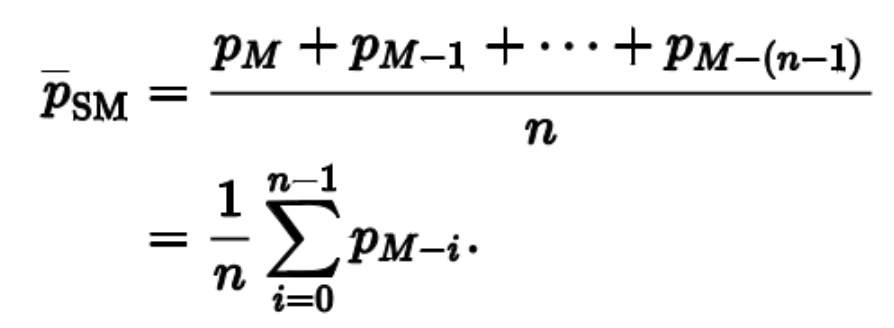### Double Moving Average Quantitative Strategy

A brief introduction In the double moving average quantitative strategy, a long-term moving average (LTMA) and a short-term moving average (STMA) are used to identify buy and sell opportunities. When the short-term moving average breaks upwards above the long-term moving average (golden cross), the strategy will be to go long. When the short-term moving average crosses downwards below the long-term moving average (death cross), the strategy will be to go short.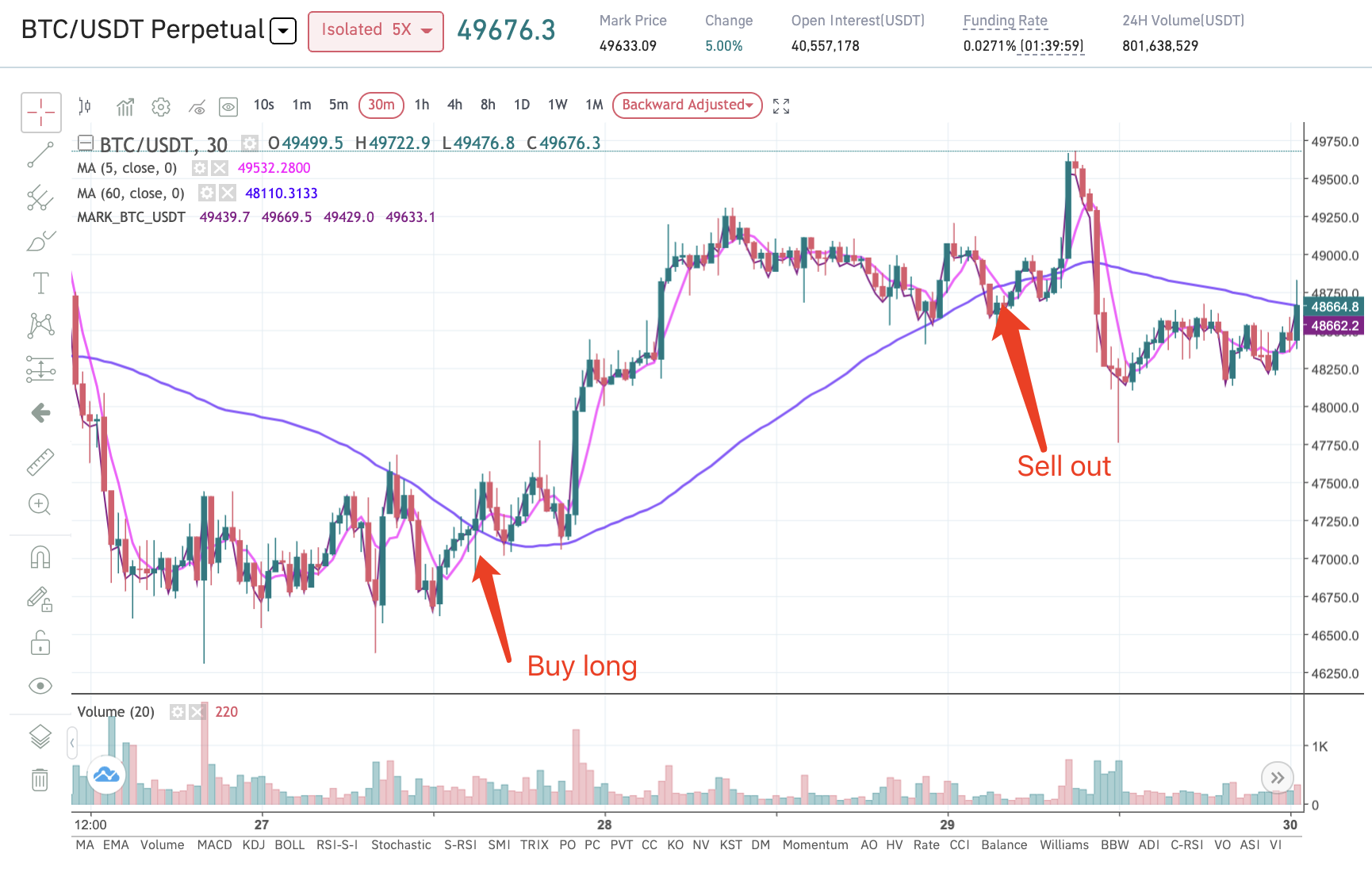### Where to set up a double moving average strategy?

(Perpetual contract double moving average as an example) Navigation bar - "Copy Trading" - "Create A New Strategy" - "Strategies Templates" - (Double Moving Average)"Create Strategy". Then select the trading market and enter the total investment amount. Click on "Create" after setting up the parameters.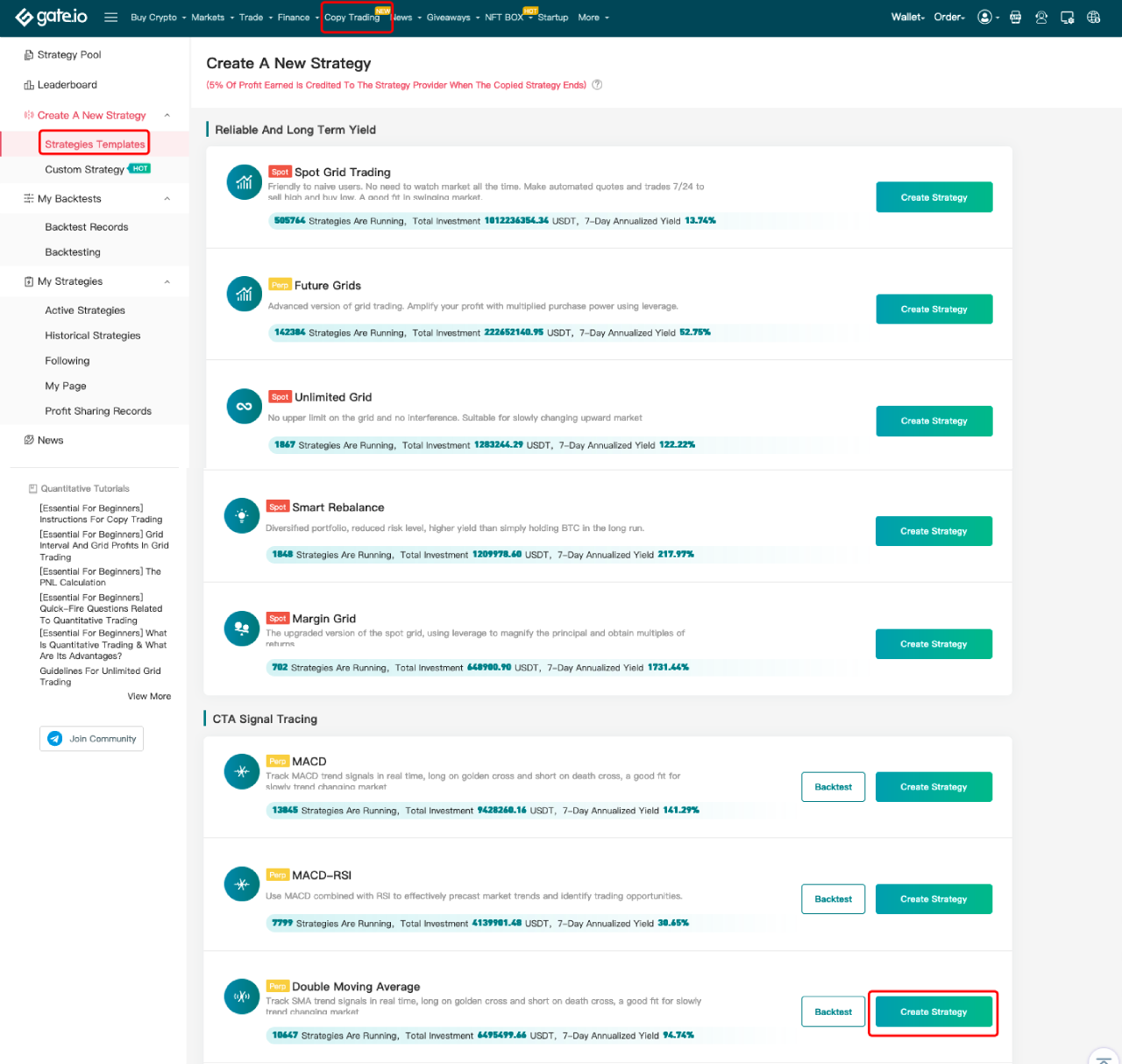Backtest : You can perform backtesting before creating double moving average strategies. The backtesting process is as follows:

Click the "Backtest" button as shown on the screenshot above. Fill in the expected parameters and click "Backtest", the will automatically backtrack the data (within one month by default), and generate a piece of backtest data for reference in the "Backtest Records".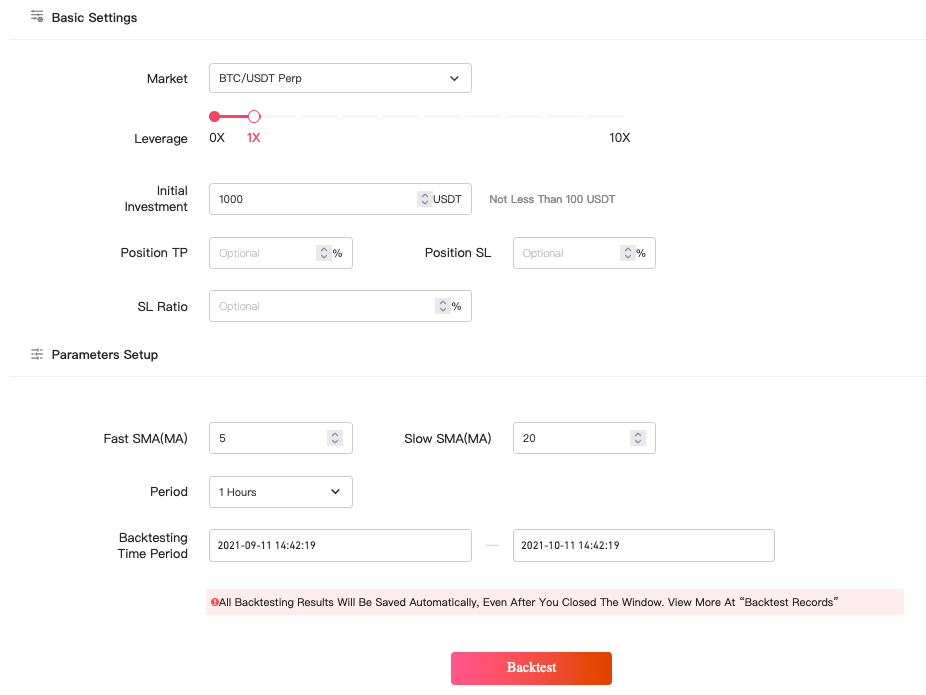### Parameters setup

######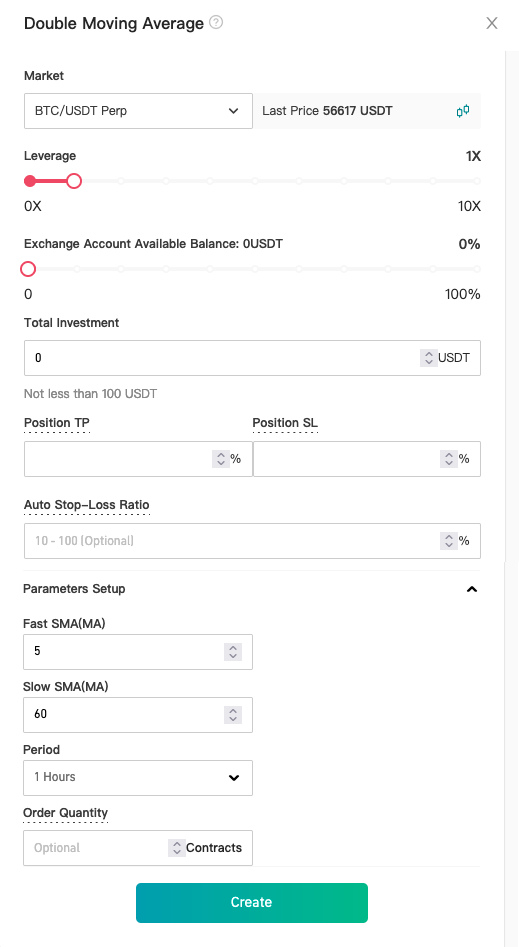Leverage ratio :The leverage ratio users choose will be used in calculating the order quantity.

Total Investment: The total amount of asset an investor puts in as margin.

Position TP : When the profit/loss ratio of the last mark price to the average opening price is equal to or greater than the position take-profit ratio, liquidation will be triggered at market price, which will not affect the ution of the strategy.

Position SL: When the profit/loss ratio of the last mark price to the average opening price is equal to or less than the position take-profit, liquidation will be triggered at market price, which will not affect the ution of the strategy.

Auto Stop-Loss Ratio: When the total investment loss reaches the auto stop-loss ratio, the strategy will close the position and exit.

Fast SMA, Slow SMA and Period : Standard parameters of MA.

Order Quantity :The number of contracts the strategy makes when a signal is triggered.

Default Order Quantity:

Default Contract Handling Fee: 0.00075

For inverse contracts:

Default order quantity = (margin * last price) / (2* 0.00075 + (1/leverage) * (1/order amount)

For linear contracts:

Default order quantity = margin/ ((2*0.00075 + 1/leverage) * last price * (1/order amount) The actual order quantity is the smaller one of the calculated default value and the value set by the investor.

Can't find the answer you want?Submit a Ticket

Popular Articles

Articles